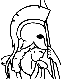#Stochastic Optimal Control: The Discrete-Time Case Table of Contents:

1. Introduction

1. Structure of Sequential Decision Problems
2. Discrete-Time Optimal Control Problems - Measurability Questions
3. The Present Work Related to the Literature

2. Monotone Mappings Underlying Dynamic Programming Models

1. Notation and Assumptions
2. Main Results
3. Application to Specific Models
1. Deterministic Optimal Control
2. Stochastic Optimal Control - Countable Disturbance Space
3. Stochastic Optimal Control - Outer Integral Formulation
4. Stochastic Optimal Control - Multiplicable Cost Functional
5. Minimax Control

3. Finite Horizon Models

1. General Results and Assumptions
2. Main Results
3. Application to Specific Models

4. Infinite Horizon Models under a Contraction Assumption

1. General Results and Assumptions
2. Convergence and Existence Results
3. Computational Methods
1. Successive Approximation
2. Policy Iteration
3. Mathematical Programming
4. Application to Specific Models

5. Infinite Horizon Models under Monotonicity Assumptions

1. General Results and Assumptions
2. The Optimality Equation
3. Characterization of Optimal Policies
4. Convergence of the Dynamic Programming Algorithm - Existence of Stationary Policies
5. Application to Specific Models

6. A Generalized Abstract Dynamic Programming Model

1. General Results and Assumptions
2. Analysis of Finite Horizon Models
3. Analysis of Infinite Horizon Models under a Contraction Assumption

7. Borel Spaces and their Probability Measures

1. Notation
2. Metrizable Spaces
3. Borel Spaces
4. Probability Measures on Borel Spaces
1. Characterization of Probability Measures
2. The Weak Topology
3. Stochastic Kernels
4. Integration
5. Semicontinuous Functions and Borel-Measurable Selection
6. Analytic Sets
1. Equivalent Definitions of Analytic Sets
2. Measurability Properties of Analytic Sets
3. An Analytic Set of Probability Measures
7. Lower Semianalytic Functions and Universally Measurable Selection

8. The Finite Horizon Borel Model

1. The Model
2. The Dynamic Programming Algorithm - Existence of Optimal and epsilon-Optimal Policies
3. The Semincontinuous Models

9. The Infinite Horizon Borel Models

1. The Stochastic Model
2. The Deterministic Model
3. Relations Between the Models
4. The Optimality Equation - Characterization of Optimal Policies
5. Convergence of the Dynamic Programming Algorithm - Existence of Stationary Optimal Policies
6. Existence of epsilon-Optimal Policies

10. The Imperfect State Information Model

1. Reduction of the Nonstationary Model - State Augmentation
2. Reduction of the Imperfect State Information Model - Sufficient Statistics
3. Existence of Sufficient Statistics for Control
1. Filtering and the Conditional Distribution of the States
2. The Identity Mappings

11. Miscellaneous

1. Limit-Measurable Policies
2. Analytically Measurable Policies
3. Models with Multiplicative Cost

12. Appendix A: The Outer Integral

13. Appendix B: Additional Measurability Properties of Borel Spaces

14. Appendix C: The Hausdorff Metric and the Exponential Topology

• References

• Index